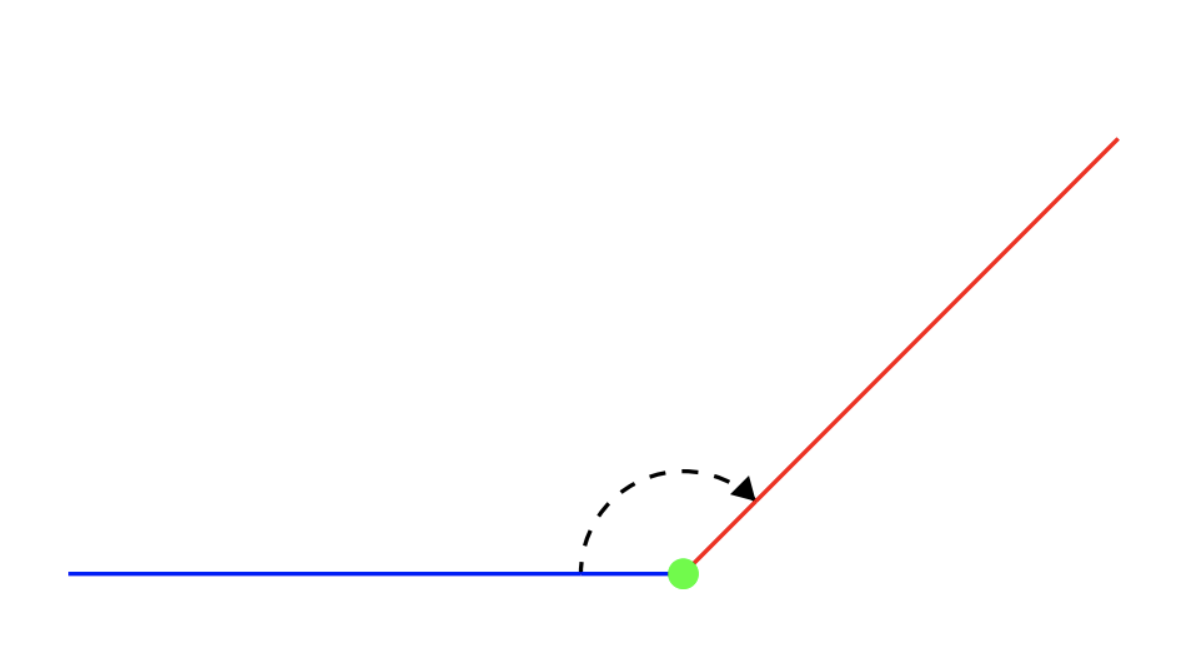# Angles

## What is an angle?

An angle is the space between two intersecting lines or surfaces at the point where they meet.## Measuring angles with a protractor

The size of an angle is measured in degrees. The symbol for degrees is °. A protractor can be used to determine the size of an angle.

You can measure from either end of a protractor, but you need to make sure that you read from the row of numbers (inner or outer) that begins with zero where the line is touching it. You also need to make sure that the point where the two lines meet is directly in the middle.

In the example above, it is the inner row of numbers that we need to look at to measure the size of this angle. This angle measures 135°.

## Drawing angles

1. Draw a straight line using a ruler. Place the centre of the protractor at the end of the line. Make sure the line passes through the zero on the protractor.

2. Find the angle you want to draw on the protractor, and make a small mark next to it. (Make sure you use the same row of numbers that includes the zero which your straight line is going through.)

3. Use the ruler to draw a straight line between the mark you have made, and the end of the first line you drew. Label the angle.

## Types of angles

An acute angle is an angle that is larger than 90º but smaller than 180º.

A right angle is 90º. Right angles are formed when two lines that are perpendicular to each intersect. For example, squares have four right angles.

An obtuse angle is an angle that is larger than 90º but smaller than 180º.

A straight angle is an angle that is 180º. A straight angle is a point on a straight line.

A reflex angle is an angle that is larger than 180º but smaller than 360º.

• When angles share a vertex and fill one side of a straight line, they add up to 180°.
• When angles share a vertex and fill the entire space around a point, they add up to 360°.
• When two lines cross, opposite angles are equal.

Angles on a straight line

a + b + c + d = 180°

Angles around a point

e + f + g = 360°

When two straight lines cross,

opposite angles are equal.

## Angles in shapes

The internal angles of a triangle always add up to 180°:

Here are internal angles of some regular polygons:

## Finding missing angles

If you have enough information about the internal angles of a shape, you can work out the size of a missing angle:

## Angles KS2

Angles are taught as part of the geometry strands of the maths curriculum. These strands are geometry - position and direction and geometry - properties of shapes.

## Angles Year 3

• recognise angles as a property of shape or a description of a turn 
• identify right angles, recognise that two right angles make a half-turn, three make three quarters of a turn and four a complete turn; identify whether angles are greater than or less than a right angle.

## Angles Year 4

• identify acute and obtuse angles and compare and order angles up to two right angles by size.

## Angles Year 5

• know angles are measured in degrees: estimate and compare acute, obtuse and reflex angles
• draw given angles, and measure them in degrees (°)
• identify:
• angles at a point and one whole turn (total 360°)
• angles at a point on a straight line and 1/2 a turn (total 180°)
• other multiples of 90°
• use the properties of rectangles to deduce related facts and find missing lengths and angles
• distinguish between regular and irregular polygons based on reasoning about equal sides and angles

## Angles Year 6

• compare and classify geometric shapes based on their properties and sizes and find unknown angles in any triangles, quadrilaterals, and regular polygons
• recognise angles where they meet at a point, are on a straight line, or are vertically opposite, and find missing angles.